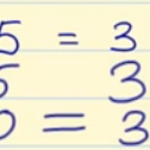Smartick is an advanced online program that teaches kids math and coding in only 15 min. a day

# I want to learn about: Mutiplication

Content about multiplication in elementary mathematics. Exercises, tutorials, problems, and teaching resources for elementary multiplication. Here you will find posts about multiplication to make learning mathematics easier and more fun.

Jun09

## Properties of MultiplicationThe properties of multiplication are distributive, commutative, associative, removing a common factor and the neutral element. We are dedicating this post to examining the properties of multiplication, which are the following: Distributive property: The multiplication of a number by a sum is equal to the sum of the multiplications of this number by each one […]